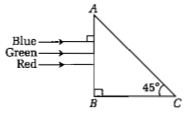The ratio of resolving powers of an optical microscope for two wavelengths  is

(a) 8:27

(b) 9:4

(c) 3:2

(d) 16:81

Concept Questions :-

Resolution of Optical devices
High Yielding Test Series + Question Bank - NEET 2020

Difficulty Level:

Two identical glass $\left({\mu }_{g}=3/2\right)$ equi-convex lenses of focal length $f$ each are kept in contact. The space between the two lenses is filled with water $\left({\mu }_{w}=4/3\right)$. The focal length of the combination is

(a)  $f/3$             (b) $f$

(c) $\frac{4f}{3}$               (d) $\frac{3f}{4}$

Concept Questions :-

Lenses
High Yielding Test Series + Question Bank - NEET 2020

Difficulty Level:

An air bubble in a glass slab with refractive index 1.5 (near normal incidence) is 5 cm deep when viewed from one surface and  3 cm deep when viewed from the opposite face. The thickness (in cm) of the slab is

(a) 8           (b) 10

(c) 12         (d) 16

Concept Questions :-

Refraction at plane surface
High Yielding Test Series + Question Bank - NEET 2020

Difficulty Level:

A person can see clearly objects only when they lie between 50 cm and 400 cm from his eyes. In order to increase the maximum distance of distinct vision to infinity, the type and power of the correcting lens, the person has to use will be-

1. convex, +2.25 diopter

2. concave, - 0.25 diopter

3. concave, - 0.2 diopter

4. convex, + 0.15 diopter

Concept Questions :-

Human eye
High Yielding Test Series + Question Bank - NEET 2020

Difficulty Level:

An astronomical telescope has an objective and eyepiece of focal lengths 40 cm and 4 cm respectively. To view an object 200 cm away from the objective, the lenses must be separated by a distance of :
(a) 46.0 cm (b) 50.0 cm
(c) 54.0 cm (d) 37.3 cm

Concept Questions :-

Telescope
High Yielding Test Series + Question Bank - NEET 2020

Difficulty Level:

Match the corresponding entries of Column 1 with Column 2. [Where m is the magnification produced by the mirror]

Column 1                         Column 2
A. m=-2                     a. Convex mirror
B. m=-1/2                  b. Concave mirror
C. m=+2                    c. Real image
D. m=+1/2                 d. Virtual Image

(a)A->a and c;B->a and d; C->a and b; D->c and d
(b)A->a and d; B->b and c; C->b and d; D-> b and c
(c)A->c and d; B->b and d;C->b and c;D->a and d
(d)A->b and c; B->b and c; C->b and d; D->a and d

Concept Questions :-

Reflection at spherical surface
High Yielding Test Series + Question Bank - NEET 2020

Difficulty Level:

The angle of incidence for a ray of light at a refracting surface of a prism is 45°. The angle of prism is 60°. If the ray suffers minimum deviation through the prism, the angle of deviation and refracting index of the material of the prism respectively are

(a)30°,$\sqrt{2}$

(b)45°,$\sqrt{2}$

(c)30°,$\frac{1}{\sqrt{2}}$

(d)45°,$\frac{1}{\sqrt{2}}$

Concept Questions :-

Prisms
High Yielding Test Series + Question Bank - NEET 2020

Difficulty Level:

Concept Questions :-

Prisms
High Yielding Test Series + Question Bank - NEET 2020

Difficulty Level:

In an astronomical telescope in normal adjustment a straight black line of length L is drawn on inside part of the objective lens. The eye-piece forms a real image of this line. The length of this image is l. The magnification of the telescope is:

(a)L/l+1

(b)L/l-1

(c)L+1/L-1

(d)L/l

Concept Questions :-

Telescope
High Yielding Test Series + Question Bank - NEET 2020

Difficulty Level:

A beam of light consisting of red, green and blue colours is incident on a right angled prism. The refractive index of the material of the prism for the above red, green and blue wavelengths are 1.39, 1.44 and 1.47, respectively.The prism will

(a) separate the blue colour part from the red and green colours

(b) separate all the three colours from one another

(c) not separate the three colours at all

(d) separate the red colour part from the green and blue colours

Concept Questions :-

Total internal reflection